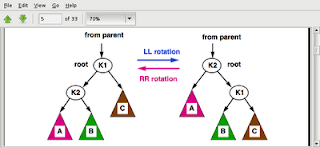Wednesday, January 14, 2009

AVL Search Tree

With BST the problem is that it should be balanced properly so that the height of a tree with node n should be log(2)n. But since BST does not have any balancing algorithm which re-balances the tree on every insert/delete, the tree becomes unbalanced. In this case the height of the tree may go up to n (same as the number of elements n). So the worst case scenario for search is O(n) for a BST.

An AVL tree on the other hand is supposed to be balanced. Balanced means that
* Either the tree is empty
* Or for every node in a non-empty tree height(left_sub_tree) - height(right_sub_tree) <= 1

The idea is that whenever we insert/remove an element from the tree, we should check that the tree is balanced and if not, we should perform "rotations" to balance the tree.

For example we have a tree

c
/
b

If we add a node "a" to the tree we will get

c
/
b
/
a

Now the left subtree is of height 2 and the right subtree is of height 0, so it is unbalanced. So a single right rotation is performed to balance the tree.

b
/ \
a   c

Lets see a few algorithms to insert and remove nodes from an AVL tree

Single LL & RR rotation will do the following converstionSimple rotation to left:

function Rotate_LL(oldRoot)
{
Result = oldRoot.left;
oldRoot.left = Result.right;
Result.right = oldRoot;
return Result;
}

Simple rotation to right:

function Rotate_RR(oldRoot)
{
Result = oldRoot.right;
oldRoot.right = Result.left;
Result.left = oldRoot;
return Result;
}

Following are the RL and LR rotations:

Double rotation to right:Double rotation to left:Lets assume that every node has a height attribute. AdjustHeight function updates the height to reflect the current height of the tree

{
if (Node != null) then
{
Node.height=1+max(height(node.left),height(node.right));
}
}

Function to get the height of the node

Function getHeight(Node)
{
if(Node == Void) return 0
else return Node.height
}

Insert record in AVL tree. Remember that the tree has to be rebalanced after insert.

Function insertData(key, data)
{
Node = new Node(key, data);
root = insertNode(root, Node);
root = rebalanceForInsert(root);
}

Function insertNode(root, newNode)
{
if (root == Void) root = newNode;
else
{
if(root.key > newNode.key)
root.left = insertNode(root.left, newNode);
else
root.right = insertNode(root.right, newNode);
}
result = rebalanceForInsert(root);
}

Function rebalanceForInsert(Node)
{
h_LL = height(Node.left.left);
h_LR = height(Node.left.right);
h_R = height(Node.right);
h_RR = height(Node.right.right);
h_RL = height(Node.right.left);
h_L = height(Node.left);
if( (h_LL == h_LR) && (h_LR >= h_R) )
result = rotate_LL(Node);
else if( (h_RR == h_RL) && (h_RL >= h_L) )
result = rotate_RR(Node);
else if( (h_LR == h_LL) && (h_LL >= h_R) )
result = rotate_LR(Node);
else if( (h_RL == h_RR) && (h_RR >= h_L) )
result = rotate_RL(Node);
else result = Node;
return result;
}

Lets look at how to remove node from an AVL tree

Function Remove(key)
{
if (root==void) result = void;
else if(root.key == key)
{
result = root.data;
root = removeNode(root);
}
else
result = removeRec(root,key);
root = rebalanceForDel(root);
}

Function RemoveRec(node, key)
{
if(root.key > key) //remove from left subtree
{
if(root.left == void) result = void;
else if(root.left.key == key)
{
result = root.left.data;
root.left = removeNode(root.left);
}
else
{
result = removeRec(root.left, key);
root.left = rebalanceForDel(root.left);
}
}
else //remove from right subtree
{
if(root.right == void) result = void;
else if(root.right.key == key)
{
result = root.right.data;
root.right = removeNode(root.right);
}
else
{
result = removeRec(root.right, key);
root.right = rebalanceForDel(root.right);
}
return result;
}

Function removeNode(Node)
{
if(Node.left == void) result = Node.right;
else if(Node.right == void) result = Node.left;
else (child = root.left)
{
if(child.right == void)
{
Node.data = child.data;
Node.left = child.left;
}
else
Node.left = swap_and_remove_left_nbr(Node,child);
result = rebalanceForDel(Node);
}
}

Function swap_and_remove_left_nbr(Parent, child)
{
if(child.right.right != void)
child.right = swap_and_remove_left_nbr(parent, child.right);
else
{
parent.data = child.right.data;
child.right = child.right.left;
}
result = rebalanceForDel(parent);
}

Function rebalanceForDel(Node)
{
h_LL = height(Node.left.left);
h_LR = height(Node.left.right);
h_R = height(Node.right);
h_RR = height(Node.right.right);
h_RL = height(Node.right.left);
h_L = height(Node.left);
if( (h_LL >= h_LR) && (h_LR >= h_R) )
result = rotate_LL(Node);
else if( (h_RR >= h_RL) && (h_RL >= h_L) )
result = rotate_RR(Node);
else if( (h_LR >= h_LL) && (h_LL >= h_R) )
result = rotate_LR(Node);
else if( (h_RL >= h_RR) && (h_RR >= h_L) )
result = rotate_RL(Node);
else result = Node;
return result;
}

That is quite a lot of stuff to digest...
Happy chewing...

1 comment:Anonymous said...

gud program...